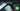# Hackerrank | Solution of Divisible Sum Pairs in Golang

October 6th, 2018
|

In this one we will solve question divisible sum pairs from hackerrank in golang. Let's get right into it.

# Problem Statement

The question can be found at this link. The problem statement states that we need to find all those pairs of numbers where sum of those numbers are divisible by a given number `k`.

# Challenges

• Choose our preferred language as golang on hackerrank. The moment we do that, we get some 50-60 lines of code which are very unfamiliar to someone who is new to language.
• Cracking the logic required to solve the question.

# Solution

This is the template you get on hackerrank for this problem statement.

``````    package main

import (
"bufio"
"fmt"
"io"
"os"
"strconv"
"strings"
)

// Complete the divisibleSumPairs function below.
func divisibleSumPairs(n int32, k int32, ar []int32) int32 {

}

func main() {

stdout, err := os.Create(os.Getenv("OUTPUT_PATH"))
checkError(err)

defer stdout.Close()

writer := bufio.NewWriterSize(stdout, 1024 * 1024)

nTemp, err := strconv.ParseInt(nk, 10, 64)
checkError(err)
n := int32(nTemp)

kTemp, err := strconv.ParseInt(nk, 10, 64)
checkError(err)
k := int32(kTemp)

var ar []int32

for i := 0; i < int(n); i++ {
arItemTemp, err := strconv.ParseInt(arTemp[i], 10, 64)
checkError(err)
arItem := int32(arItemTemp)
ar = append(ar, arItem)
}

result := divisibleSumPairs(n, k, ar)

fmt.Fprintf(writer, "%d\n", result)

writer.Flush()
}

if err == io.EOF {
return ""
}

return strings.TrimRight(string(str), "\r\n")
}

func checkError(err error) {
if err != nil {
panic(err)
}
}``````
• First go ahead and delete everything in the pre-existing template.
• Let's write down our main wrapper function.
``````package main
import "fmt"

func main(){

}``````

Let's discuss the variables that we would need for our question. We need the length of array `n`, the number `k`, the counter the store the result `c`, and the array itself. Since we need to find pairs of numbers, instead of taking inputs in temporary variables we would need it in a array so that we can loop over it and form a pair. Let's declare our variables, our array, takes inputs and come back again to discuss the logic.

``````	...
var n,k,c int
fmt.Scan(&n,&k)

a := make([]int,n)

for i:=0;i<n;i++ {
fmt.Scan(&a[i])
}
``````

Nothing fancy here, declaring the variables as discussed, declaring the array and taking it's elements as inputs. Let's discuss the logic now. So we need to form pairs in a way where the `a[i]+a[j] % k = 0 and i<j` , so it's pretty simple we will have an outer loop which will loop over the array from `0` to length of array while the inner loop will loop over the array from `i+1` i.e. the condition that `j>i` to length of array. Now we can form pairs of numbers from `(i,j) index` and check for divisibility by `k`. If it is divisible, we will increase the counter, else we will move one in the next loop. If that sounds clear, let's implement it now.

``````	...
for i:=0;i<n;i++{
for j:=i+1;j<n;j++{
if (a[i]+a[j]) % k == 0 {
c++
}
}
}
fmt.Println(c)``````

Just implementing the logic we discussed and printing our counter. Now your complete code should look like

``````package main

import "fmt"

func main(){
var n,k,c int
fmt.Scan(&n,&k)

a := make([]int,n)

for i:=0;i<n;i++ {
fmt.Scan(&a[i])
}

for i:=0;i<n;i++{
for j:=i+1;j<n;j++{
if (a[i]+a[j]) % k == 0 {
c++
}
}
}
fmt.Println(c)
}``````

This was a quick and easy one. This is it for this one, complete source code for this post can be found on my Github Repo. Will see you in the next one.